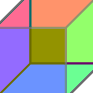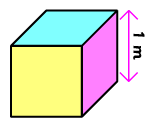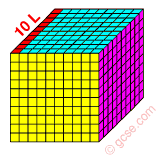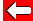# Cubic MetresOne cubic metre (1 m³) is a cube where each edge is 1 m long:We have seen that 1 litre is a cube 10 cm along each edge. We would be able to fit 10 L along each edge, 100 L on each face, and 1,000 L inside the whole cubic metre:Another way of looking at it is that 1 m³ is 100 cm along each edge, so its volume will be 100 × 100 × 100 = 1,000,000 cm³Go back a pageMaths MenuGo to next page